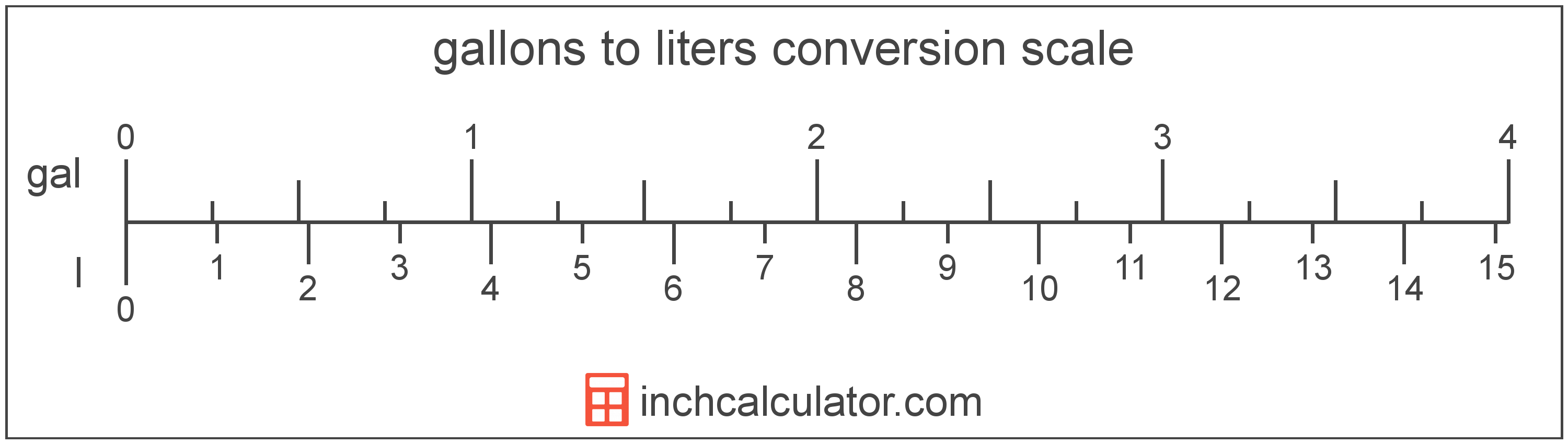Convert Gallons to Liters

Enter the volume in gallons below to get the value converted to liters.

Results in Liters:1 gal = 3.785412 l
Do you want to convert liters to gallons?

How to Convert Gallons to LitersTo convert a gallon measurement to a liter measurement, multiply the volume by the conversion ratio. One gallon is equal to 3.785412 liters, so use this simple formula to convert:

liters = gallons × 3.785412

The volume in liters is equal to the gallons multiplied by 3.785412.

For example, here's how to convert 5 gallons to liters using the formula above.
5 gal = (5 × 3.785412) = 18.927059 l

Gallons and liters are both units used to measure volume. Keep reading to learn more about each unit of measure.

Gallons

The US liquid gallon is a unit of fluid volume equal to four quarts, eight pints, or sixteen cups. The US liquid gallon should not be confused with the US dry gallon or the imperial gallon, which are different units of measure.

The gallon is a US customary unit of volume. Gallons can be abbreviated as gal, for example 1 gallon can be written as 1 gal.

Liters

The liter is a unit of volume equal to 1,000 cubic centimeters. The liter is a special name defined for the cubic decimeter, and is exactly equal to the volume of one cubic decimeter.

The liter is an SI accepted unit for volume for use with the metric system. A liter is sometimes also referred to as a litre. Liters can be abbreviated as l, and are also sometimes abbreviated as L or . For example, 1 liter can be written as 1 l, 1 L, or 1 ℓ.

Gallon Measurements and Equivalent Liter Conversions

Common gallon values converted to the equivalent liter value
Gallons Liters
1 gal 3.7854 l
2 gal 7.5708 l
3 gal 11.36 l
4 gal 15.14 l
5 gal 18.93 l
6 gal 22.71 l
7 gal 26.5 l
8 gal 30.28 l
9 gal 34.07 l
10 gal 37.85 l
11 gal 41.64 l
12 gal 45.42 l
13 gal 49.21 l
14 gal 53 l
15 gal 56.78 l
16 gal 60.57 l
17 gal 64.35 l
18 gal 68.14 l
19 gal 71.92 l
20 gal 75.71 l
21 gal 79.49 l
22 gal 83.28 l
23 gal 87.06 l
24 gal 90.85 l
25 gal 94.64 l
26 gal 98.42 l
27 gal 102.21 l
28 gal 105.99 l
29 gal 109.78 l
30 gal 113.56 l
31 gal 117.35 l
32 gal 121.13 l
33 gal 124.92 l
34 gal 128.7 l
35 gal 132.49 l
36 gal 136.27 l
37 gal 140.06 l
38 gal 143.85 l
39 gal 147.63 l
40 gal 151.42 l

More Gallon Volume Conversions

US Liquid
Convert to Quarts
1 gal is equal to 4 quarts
Convert to Pints
1 gal is equal to 8 pints
Convert to Fluid Ounces
1 gal is equal to 128 fluid ounces
US Cooking
Convert to Cups
1 gal is equal to 16 cups
Convert to Tablespoons
1 gal is equal to 256 tablespoons
Convert to Teaspoons
1 gal is equal to 768 teaspoons
US Customary Volume
Convert to Cubic Inches
1 gal is equal to 231 cubic inches
Convert to Cubic Feet
1 gal is equal to 0.133681 cubic feet
Convert to Cubic Yards
1 gal is equal to 0.004951 cubic yards
Metric Volume
Convert to Milliliters
1 gal is equal to 3,785.41 milliliters
Convert to Cubic Centimeters
1 gal is equal to 3,785.41 cubic centimeters
Convert to Cubic Meters
1 gal is equal to 0.003785 cubic meters